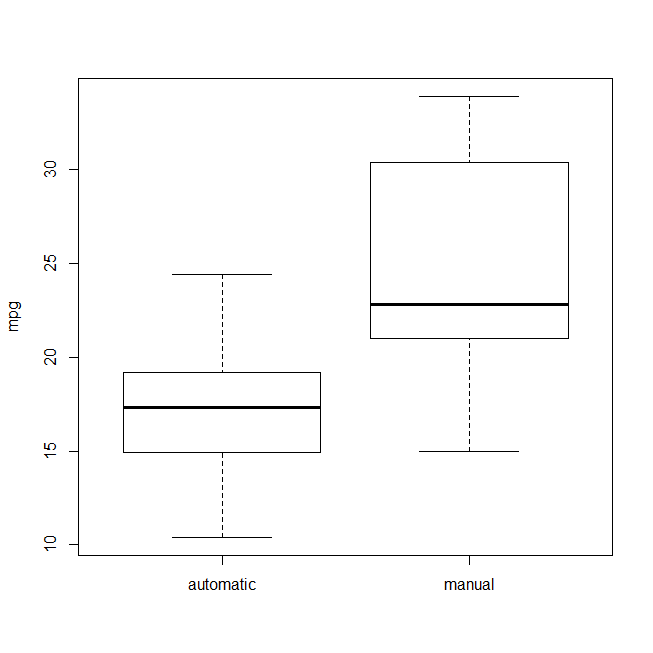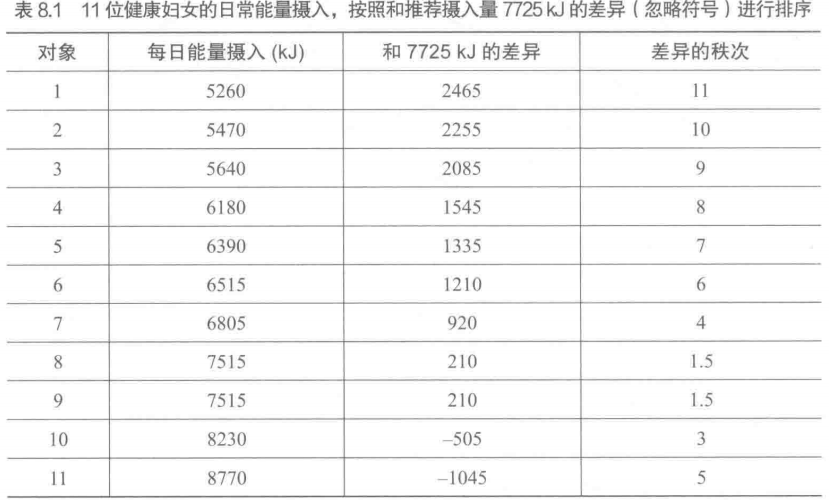• ## Wilcoxon 检验之 rank-sum 与 signed-rank

万次阅读 多人点赞 2018-08-31 18:26:06
Frank Wilcoxon 是美国的统计学家，终其一生最著名的就是提出了 2 个非参假设检验方法，即 秩和检验 (Wilcoxon rank-sum test) 和 符号秩检验 (wilcoxon signed-rank test)。本文简单的对比总结了两种方法的用法，...
前些时候在写作时碰到了 Wilcoxon 检验，仔细一查，发现这里面居然还包含 2 种不同类型的检测，并且极容易混淆，这 2 种分别方法是 Wilcoxon rank-sum test（我翻译为秩和检验）和 Wilcoxon signed-rank test（我翻译为符号秩检验）。今天我简单总结一下，对比一下他们的差异。Frank Wilcoxon (1892—1965) 是美国的统计学家，发表了 70 篇左右论文，但其最大的贡献就是这 2 个以他名字命名的非参假设检验方法：秩和检验 和 符号秩检验。他在 1945 年发表的论文 1 中将二者分别称为 非成对检验 （unpaired experiment）和 成对检验（paired comparison）。 正是因为其巨大影响力使得这两个检验方法都以他的名字命名，并流传下来。
假设检验有点类似于我们高中数学中常见的“反证法”，即提出一个错误的假设，然后证明它是错的。那么我们提出的假设叫做 原假设 (Null Hypothesis)，简写为 $H_{0}$。我们备选的假设叫做 备选假设 (Alternative Hypothesis)，简写为 $H_{\alpha}$ 或者 $H_{1}$。注意，在假设检验中只有 2 个假设，即原假设和备选假设，我们的目的就是要拒绝原假设。
在假设检验过程中，我们一般设定原假设 $H_0$ 和备选假设 $H_1$ 如下，

原假设 $H_0$：两组数据没有显著性差异
备选假设 $H_1$：两组数据存在显著性差异

1. Wilcoxon 秩和检验
根据 wikipedia 解释， Wilcoxon rank-sum test 定义如下，

In statistics, the Mann–Whitney U test (also called the Mann–Whitney–Wilcoxon (MWW), Wilcoxon rank-sum test, or Wilcoxon–Mann–Whitney test) is a nonparametric test.
This test can be used to determine whether two independent samples were selected from populations having the same distribution. 2

基本概念： 在统计学中，Wilcoxon rank-sum test（威尔科克森秩和检验）也叫 Mann-Whitney U test（曼-惠特尼 U 检验），或者 Wilcoxon-Mann-Whitney test。秩和检验是一个非参的假设检验方法，一般用来检测 2 个数据集是否来自于相同分布的总体。
这里的 “秩” 其实就是 “排名” 的意思，“秩和” 当然就是指 “将排名进行求和” 的操作。在秩和检验中，我们不要求被检验的 2 组数据包含相同个数的元素，换句话说，秩和检验更适用于非成对数据之间的差异性检测。
应用实例： 假设我们有 2 组数据 $x_{1}$ 和 $x_{2}$，如下表所示，$x_{1}$ 中有 7 个元素（列 $x_1$ 中），$x_{2}$ 中有 8 个元素（列 $x_2$ 中），现在使用秩和检验判断这 2 组数据是否存在显著性差异。

数据 $x_{1}$
总排名 $rank$
数据$x_{2}$
总排名 $rank$

9
14
7
11

5
5.5
4
3

8
13
5
5.5

7
11
6
8

10
15
3
1

6
8
6
8

7
11
4
3

4
3

步骤 1：我们首先将 $x_{1}$ 和 $x_{2}$ 整合成一个序列，并按升序重新排序，序号记在表中的 $rank$ 列当中。我们分别计算 2 组数据的排名之和 $R_{1}$ 和 $R_{2}$ 有，
$R_{1} = (14+5.5+13+11+15+8+11) = 77.5$ $R_{2} = (11+3+5.5+8+1+8+3+3) = 42.5$
注意，当我们计算若干等值元素的排名时，会用这些元素排名的平均值作为它们在整个序列中的排名。例如，$x_1$ 中的第 2 个元素与 $x_2$ 中第3 个元素的值都等于 5，且这 2 个 5 在整个序列中的排名分别是第 5 和第 6，因此这两个元素的排名为 $\frac{5+6}{2}=5.5$。其余等值元素的排名计算也与之类似。
步骤 2：令 $n_{1}$ 和 $n_{2}$ 分别表示 2 组数据的个数，即 $n_{1}=7, n_{2}=8$。再令 $T$ 表示小样本的排名和，即 $T = R_{1} = 77.5$。根据计算公式可得 $U_{1}$ 和 $U_{2}$ 的值如下，
$U_{1} = n_{1} \times n_{2} + \frac{n_{1}(n_{1}+1)}{2} - T = 7\times8 + \frac{7\times8}{2} - 77.5 = 6.5$ $U_{2} = n_{1} \times n_{2} - U_{1} = 7 \times 8 - 6.5 = 49.5$
步骤 3：由于 $U_{1}$ 更小，我们依此来查 Wilcoxon 双尾临界表，当 $\alpha=0.05, n_{1} = 7, n_{2} = 8$ 时的临界值是 10。因为 $U_{1} < 10$，故应该拒绝原假设。最终结论是：$x_{1}$ 和 $x_{2}$ 存在统计意义上的显著性差异，它们可能来自分布不同的总体。
编程实现： 在 python 中我们调用 scipy 包来里的 stats.mannwhitneyu() 函数来实现秩和检验，如下代码，
from scipy import stats
def wilcoxon_rank_sum_test(x, y):
res = stats.mannwhitneyu(x ,y)
print(res)

得到的结果如下，可知 statistic 即我们的 $U_{1}$ 值 $U_{1}$ = statistic = 6.5，pvalue 即我们的 p-value 值 pvalue=0.0069…
> MannwhitneyuResult(statistic=6.5, pvalue=0.006966479792405637)

2. Wilcoxon 符号秩检验
根据 wikipedia 解释， Wilcoxon signed-rank test 定义如下，

A Wilcoxon signed-rank test is a nonparametric test that can be used to determine whether two dependent samples were selected from populations having the same distribution. 3

基本概念： Wilcoxon signed-rank test（威尔科克森符号秩检验）也是一种非参的假设检验方法，它成对的检查 2 个数据集中的数据（即 paired difference test）来判断 2 个数据集是否来自相同分布的总体。
应用实例： 假设我们有 2 组数据 $y_{1}$ 和 $y_{2}$，如下表所示。我们按照如下 3 步来计算 wilcoxon signed-rank test 的结果。

$ID$
数据 $y_{1}$
数据 $y_{2}$
符号位 $sign$
绝对差值 $abs$
绝对差值的排名 $rank$

0
125
110
+1
15
7

1
115
122
-1
7
3

2
130
125
+1
5
1.5

3
140
120
+1
20
9

4
140
140
-
0
-

5
115
124
-1
9
4

6
140
123
+1
17
8

7
125
137
-1
12
6

8
140
135
+1
5
1.5

9
135
145
-1
10
5

步骤 1：首先对 $y_{1}$ 和 $y_{2}$ 两两成对配对形成 10 个数据对（即 $ID=0,...,9$），然后将这 10 个数据对两两求差，得到符号位 $sign$ 列。具体的做法是：当 $y_1$ 元素比 $y_2$ 对应元素大时，符号位为正，即 +1；当 $y_1$ 元素比 $y_2$ 对应元素小时，符号位为负，即 -1。例如，在 $ID=1$ 的数据对中，125 > 110，故其符号位为 +1.
步骤 2： 首先对 $y_{1}$ 和 $y_{2}$ 两两成对求差得到绝对值 $abs$ 列，然后根据 $abs$ 列排序得到 $rank$ 列。当某一对 $y_{1}$ 和 $y_{2}$ 的元素相等时，即 $abs=0$ 时，我们不计算其 $rank$ 值。例如，在 $ID=4$ 的数据对中，$y_1$ 和 $y_2$ 的值都是 140，因此这对数组没有排名值。
步骤 3： 有了这个 $sign$ 和 $rank$ 列的结果后，我们就可以来计算秩和了，其中大于 0 的秩和 |$W^{+}$| 和 对于小于 0 的秩和 |$W^{-}$|，以及最终的符号秩和 |$W$| 如下所示，
$|W^{+}| = |7+1.5+9+8+1.5| = 27$
$|W^{-}| = |3+4+6+5| = 18$
$|W| = ||W^{+}|-|W^{-}|| = 9$
步骤 4：最后我们根据 |$W$| 来查表 4，我们得到当 Wilcoxon 在 $\alpha=0.05$ $n=9$ 的时候的临界值是 5，而我们计算出来的 $|W| = 9 > 5$，因此我们不能拒绝原假设。最终结论是：$y_{1}$ 和 $y_{2}$ 不存在统计意义上的显著性差异，它们可能来自于同一分布的总体。
编程实现： 在 python 中我们调用 scipy 包来里的 stats.wilcoxon() 函数来实现秩和检验，如下代码，
from scipy import stats
def wilcoxon_signed_rank_test(y1, y2):
res = stats.wilcoxon(y1, y2)
print(res)

得到的结果如下，其中 statistic = 18.0，表示 2 类符号秩和较小的一个（$|W^{+}|$ 和 $|W^{-}|$ 最小的是18）；pvalue=0.5936… 就是我们需要的 p-value 值。之所以出现 Warning 信息是因为我们的数据量太少，一般来讲大于 20 是比较合适做假设检验的。
> E:\Software\Anaconda2\lib\site-packages\scipy\stats\morestats.py:2397: UserWarning:
>   Warning: sample size too small for normal approximation.
>   warnings.warn("Warning: sample size too small for normal approximation.")
> WilcoxonResult(statistic=18.0, pvalue=0.5936305914425295)


Wilcoxon, Frank. “Individual Comparisons by Ranking Methods.” Biometrics Bulletin 1.6(1945):80-83. link ↩︎

Wikipedia. Wilcoxon rank-sum test. link ↩︎

Wikipedia. Wilcoxon signed-rank test. link ↩︎


展开全文• 用于评估配对（相关）样本之间差异的非参数 Wilcoxon 检验。 如果差值小于15，则算法计算精确的秩分布； 否则它使用正态分布近似。 现在，MatLab 函数 SIGNRANK 返回相同的 p 值。 无论如何，这个 Wilcoxon 函数提供...matlab
• 此脚本有助于研究对从具有不同上端点的连续均匀分布生成的人工数据的配对 Wilcoxon 符号秩检验的属性。 模型的参数在“实验参数初始化”部分定义和描述。 该脚本生成 3 个伪彩色图，它们是： * Wilcoxon 符号秩...matlab
• https://stats.stackexchange.com/questions/91034/difference-between-the-wilcoxon-rank-sum-test-and-the-wilcoxon-signed-rank-test 1. You should use the signed rank test when the data arepaired. You'....
https://stats.stackexchange.com/questions/91034/difference-between-the-wilcoxon-rank-sum-test-and-the-wilcoxon-signed-rank-test

1.

You should use the signed rank test when the data are paired.

You'll find many definitions of pairing, but at heart the criterion is something that makes pairs of values at least somewhat positively dependent, while unpaired values are not dependent. Often the dependence-pairing occurs because they're observations on the same unit (repeated measures), but it doesn't have to be on the same unit, just in some way tending to be associated (while measuring the same kind of thing), to be considered as 'paired'.

You should use the rank-sum test when the data are not paired.

That's basically all there is to it.

Note that having the same nn doesn't mean the data are paired, and having different nn doesn't mean that there isn't pairing (it may be that a few pairs lost an observation for some reason). Pairing comes from consideration of what was sampled.

The effect of using a paired test when the data are paired is that it generally gives more power to detect the changes you're interested in. If the association leads to strong dependence*, then the gain in power may be substantial.

* specifically, but speaking somewhat loosely, if the effect size is large compared to the typical size of the pair-differences, but small compared to the typical size of the unpaired-differences, you may pick up the difference with a paired test at a quite small sample size but with an unpaired test only at a much larger sample size.

However, when the data are not paired, it may be (at least slightly) counterproductive to treat the data as paired. That said, the cost - in lost power - may in many circumstances be quite small - a power study I did in response to this question seems to suggest that on average the power loss in typical small-sample situations (say for n of the order of 10 to 30 in each sample, after adjusting for differences in significance level) may be surprisingly small.

[If you're somehow really uncertain whether the data are paired or not, the loss in treating unpaired data as paired is usually relatively minor, while the gains may be substantial if they are paired. This suggests if you really don't know, and have a way of figuring out what is paired with what assuming they were paired -- such as the values being in the same row in a table, it may in practice may make sense to act as if the data were paired to be safe -- though some people may tend to get quite exercised over you doing that.]

2.

I'm not a researcher, I'm a statistics major though. I'll first layout the requirements for the Wilcoxon Signed Rank Sum Test (WSRST).

The WSRST requires that the populations be paired, for example, the same group of people are tested on two different occasions or things and MEASURED on the effects of each and we then compare the two things or occasions.
The WSRST requires the data to be quantitative. Quantitative data is data that is measured along a scale, that is why I highlighted the world measured in the first point. Had the participants been asked to rank their responses, you will then be dealing with qualitative data, where you will then have to use the sign test to test your hypothesis.
[There are other requirements for the WSRST but the ones I've listed are sufficient to differentiate the two tests]

Now the Wilcoxon Rank Sum Test (WRST)

The main requirement is that the samples be drawn from independent populations. For example you might want to test whether the exam paper 1 is harder than exam paper 2, and to do this you will have two groups of students, and the groups need not be the same size. From the example the two groups are independent, if you had asked the same group to write the same paper twice, then you will use the WSRST to test your hypothesis.
The other requirement is that the data need not be quantitative, i.e. you can also perform the test on qualitative data.

展开全文• 说明wilcoxon秩和及wilcoxon符号秩检验是对原假设的非参数检验，在不需要假设两个样本空间都为正态分布的情况下，测试它们的分布是否完全相同。
说明

wilcoxon秩和及wilcoxon符号秩检验是对原假设的非参数检验，在不需要假设两个样本空间都为正态分布的情况下，测试它们的分布是否完全相同。

操作

#利用mtcars数据
library(stats)
data("mtcars")
boxplot(mtcars$mpg~mtcars$am,ylab='mpg',names = c('automatic','manual))#执行wilcoxon秩和检验验证自动档手动档数据分布是否一致

wilcox.test(mpg~am,data = mtcars)

#wilcox.test(mtcars$mpg[mtcars$am==0],mtcars$mpg[mtcars$am==1])（与上面等价）
Wilcoxon rank sum test with continuity correction

data:  mpg by am
W = 42, p-value = 0.001871
alternative hypothesis: true location shift is not equal to 0

Warning message:
In wilcox.test.default(x = c(21.4, 18.7, 18.1, 14.3, 24.4, 22.8,  :
无法精確計算带连结的p值


总结

执行wilcoxon秩和检验（也称Mann-Whitney U检验）这样一种非参数检验   。t检验假设两个样本的数据集之间的差别符合正态分布（当两个样本集都符合正态分布时，t检验效果最佳），但当服从正态分布的假设并不确定时，我们执行wilcoxon秩和检验来验证数据集中mtcars中自动档与手动档汽车的mpg值的分布是否一致，p值<0.05,原假设不成立。意味两者分布不同。警告“无法精確計算带连结的p值“这是因为数据中存在重复的值，一旦去掉重复值，警告就不会出现。
展开全文r语言
• 提出一种基于最小Wilcoxon学习方法的非线性动态系统建模方法。用非线性静态子环节和线性动态子环节串联——Hammerstein模型来描述非线性动态系统。然后,将Hammerstein模型的非线性传递函数转换为等价的线性形式,从而...
• 我们可以进行Wilcoxon符号秩和检验。注意和单样本t检验不同，该检验检查是否有差异： (rank, pVal) = stats.wilcoxon(data-checkValue) 该方法有3个步骤： 计算每个观测值和感兴趣的值的差异； 忽略差异的符号，将...
在非正态分布的数据中，我们不应该使用单样本t检验（尽管这个检验对于偏离正态性相当稳健），相反，我们必须使用均值的非参数检验方法。我们可以进行Wilcoxon符号秩和检验。注意和单样本t检验不同，该检验检查是否有差异：
(rank, pVal) = stats.wilcoxon(data-checkValue)

该方法有3个步骤：

计算每个观测值和感兴趣的值的差异；
忽略差异的符号，将他们按照大小排序；
将所有负（或正）秩次的秩次加起来，也就是那些低于（或高于）选定的假设值的秩次。

在下面的表格中，你可以看到一个判断是否显著偏离7725的检验，负值的秩次之和为3+5=8，并且可以在对应的表格中找到显著的，在实际中，你的python函数语句会帮你做这些。
这个例子也展示了秩次求值的另外一个特征：相同的值（在这里是7515）得到的是它们的平均秩次展开全文python 数据分析
• 执行 Wilcoxon 秩和检验或 Mann-Whitney U 检验（非参数检验）。 测量健康的基因/化合物/错误/代谢物等（对照）个体和患有某种疾病的个体。 然后进行 Wilcoxon 秩和检验，以找出这些基因/化合物/代谢物/错误等中有...matlab
• 基于WILCOXON秩和检验和绝对偏差的遥感图像分割。研究论文
• 此函数为一组三个或更多独立随机样本的趋势提供 Wilcoxon 型检验。 假设： 数据必须至少是有序的 必须以有意义的顺序选择组，即排序 如果您不选择输入自己的组分数，则分数将按照 n 组的选择顺序统一分配 (1 ... n)...matlab
• Wilcoxon Research推出一种低成本通用加速计，敏感度达100mV/g，公差为10%。788A为单轴传感器，具有按条件监测和预测维护程序，性价比高，加速范围为±50g，适合监视风扇、电机、泵、吹风机、压缩机、冷却器、变速箱...
• 介绍用 Excel进行配对Wilcoxon符号检验的方法和工具
• ## Wilcoxon Signed-Rank Test

千次阅读 2018-09-23 14:56:01
Wilcoxon Signed-Rank Test using SPSS Statistics
• 前一篇文章我们介绍了秩和检验，这里我们介绍另一种秩和检验方法，Wilcoxon signed rank sum test 文章目录 Wilcoxon signed rank sum test包含两种检验方式，数据成对的情况和数据是独立观察的情形。下面分开讨论...数据分析
• ## Wilcoxon符号秩检验

千次阅读 2018-08-14 11:52:36
Wilcoxon符号秩检验  它适用于T检验中的成对比较，但并不要求成对数据之差服从正态分布，只要求对称分布即可。检验成对观测数据之差是否来自均值为0的总体(产生数据的总体是否具有相同的均值)。 在Matlab中，秩和...
• Wilcoxon秩和检验(rank-sum test)，有时也叫Mann-Whitney U检验，是另一类非参数检验方法，它们不对数据分布作特殊假设，因而能适用于更复杂的数据分布情况。 适用性 （1）资料的总体分布类型未知； （2）资料的总体...机器学习 Matlab
• Wilcoxon 符号秩检验的检验目的和符号检验是一样的，但 Wilcoxon 符号秩检验需要假设样本点来自连续对称总体分布，在这个假设下总体的对称中心是总体中位数之一。Wilcoxon 符号秩检验就是要检验双边问题 H0 ：M = M0...python 非参数统计
• Wilcoxon Research推出一种低成本通用加速计，敏感度达100mV/g，公差为10%。788A为单轴传感器，具有按条件监测和预测维护程序，性价比高，加速范围为±50g，适合监视风扇、电机、泵、吹风机、压缩机、冷却器、变速箱...
• 在这篇文章中，我们将探索比较两组依赖（即成对）定量数据的测试：Wilcoxon符号秩检验和配对学生t检验。这些测试之间的关键区别在于Wilcoxon的测试是非参数测试，而t测试是参数测试。在下文中，我们将探讨这种差异的...R语言
• Wilcoxon signed-rank test(Wilcoxon符号秩检验)是非参数统计中符号检验法的改进，不仅利用了观测值和原假设中心位置的差的正负，还利用了差的值的大小的信息。虽然是简单的非参数方法，但却体现了秩的基本...
• python威氏符号秩次检验（Wilcoxon Signed-Rank Test） 1、Wilcoxon Signed Rank Test Wilcoxon有符号秩检验(也称为Wilcoxon有符号秩和检验)是一种非参数检验。当统计数据中使用“非参数”一词时，并不...数据挖掘 机器学习 深度学习 人工智能 自然语言处理
• R符号秩检验（WILCOXON SIGNED RANK TEST） 目录 R符号秩检验（WILCOXON SIGNED RANK TEST） 假设检验 假设检验的应用 符号秩检验 符号秩检验结果解读 假设检验 假设检验使用统计学中的概念来...R 机器学习 大数据 数据分析 人工智能
• Wilcoxon有符号秩检验(也称为Wilcoxon有符号秩和检验)是一种非参数检验。当统计数据中使用“非参数”一词时，并不意味着您对总体一无所知。这通常意味着总体数据没有正态分布。如果两个数据样本来自重复观察，那么...
• Wilcoxon 符号秩检验 威尔科克森符号秩检验亦称威尔科克伦代符号的等级检验，是由威尔科克森（F·Wilcoxon）于1945年提出的。该方法是在成对观测数据的符号检验基础上发展起来的，比传统的单独用正负号的检验更加...统计学 python matlab
• Wilcoxon signed-rank是一种非参数检验的统计量，用于检验对称分布的均值是否为0。给出iid数据Y1,⋯ ,YnY_1,\cdots,Y_nY1​,⋯,Yn​，Zj=sign(Yj)Z_j = sign(Y_j)Zj​=sign(Yj​)，RjR_jRj​为ZjZ_jZj​的秩(rank)....统计学 统计模型
• nestedRanksTest包提供了使用嵌套秩对处理水平之间的差异执行 Mann-Whitney-Wilcoxon 型非参数检验的函数，以及显示检验结果的函数。 当观察被分成几个组并且每个组都接受了两个处理级别时，可以使用嵌套等级测试。 ...HTML
• 该文件执行非参数 Mann-Whitney-Wilcoxon 检验来评估未配对样本之间的差异。 如果组合数小于20000，算法计算出准确的秩分布； 否则它使用正态分布近似。 结果跟不一样RANKSUM MatLab函数，但有更多输出信息。 该测试...matlab
• R语言详解参数检验和非参数检验二、参数检验R语言实现2.1 单样本t检验2.2 独立样本t检验2.3 配对样本t检验2.4方差分析2.5 pearson相关性检验三、非参数检验R语言实现3.1单样本wilcoxon检验3.2 Mann-Whitney检验3.3...数据分析 机器学习 数据挖掘
• Student t检验（Student’s t test），亦称T检验，是用t分布理论来推论差异发生的概率，从而比较两个平均数的差异是否显著，主要用于样本含量较小...Wilcoxon秩和检验（Wilcoxon rank-sum test），也叫曼-惠特尼U......